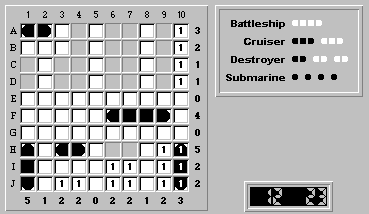Here's an example of submitting a Fathom It! board solution by e-mail:

Following is the solution for Intermediate board #10.

Finalizing (A,1) as the obvious left ship segment enables us to finalize (H,4) as a right ship segment: <DIAGRAM (ed. this instructs the editor to insert a board diagram at this point)>

The battleship can only be positioned within the squares (F,6) to(F,10). Wherever it is placed, the squares (F,7), (F,8) and (F,9) are ship segments. We also know that the remaining unknown squares in row F, (F,1/3/4), are water. This results in: <DIAGRAM>

Let's concentrate on placing the two cruisers. At first glance, there are four possible positions for a cruiser:

(A,10)-(C,10)
(B,10)-(D,10)
(H,1)-(J,1)
(H,10)-(J,10)

We can immediately rule out (B,10)-(D,10), as this would turn squares (C,1) and (D,1) into water, leaving too few squares in column 1 for ship segments.

The cruiser positions (A,10)-(C,10) and (H,10)-(J,10) are mutually exclusive; although either can hold a cruiser, column 10 can only take three ship segments.

Therefore, a cruiser must reside in the remaining position (H,1)-(J,1): <DIAGRAM>

There are only two possible positions for the last cruiser:(A,10)-(C,10) and (H,10)-(J,10). No matter which is correct, square (F,10) will be water. Therefore, the square (F,6) is the left segment of the battleship: <DIAGRAM>

We'll now show that a cruiser cannot be placed in position (H,10)-(J,10). The following figure shows what the board would look like if a cruiser were placed at (H,10)-(J,10):

1 2 3 4 5 6 7 8 9 0
A L R W W W w 3
B W W W W W w 2
C W W W w 1
D W W W w 1
E W W W W W W W W W W 0
F W W W W W L M M R W 4
G W W W W W W W W W W 0
H T W L R W W w t 5
I M W W W W w w W w m 2
J B W w w W w w W w b 2
5 1 2 2 0 2 2 1 2 3

 Editor's note: The textual board above is the equivalent of the graphic board below.The legend is:L = LeftM = MiddleR = RightT = TopB = BottomW = WaterA = Anypiece (wildcard)Uppercase letters are finalized segmentsLowercase letter are unfinalized guessesThe letter and numbers around the board are the coordinates and row/col tallies.

Because row H is missing one remaining ship segment, a submarine must be placed in either (H,6) or (H,7). However, because columns 6 and 7 are perfectly symmetrical, the submarine can be placed at either (H,6) or (H,7) without changing the rest of the solution. This would mean that there are two perfectly acceptable, and ambiguous, solutions for this board. Because Fathom It! boards are guaranteed to have a unique solution, a cruiser cannot be placed at (H,10)-(J,10). The remaining cruiser must be at (A,10)-(C,10). Finalizing a cruiser at (A,10)-(C,10) gives us: <DIAGRAM>

Finalizing the forced ship segments beginning with (D,1), and noting that the remaining destroyer can only be located at (H,6)-(H,7), leads us to the solution: <DIAGRAM>